Courses

# The Direct Stiffness Method: Truss Analysis - 3 GATE Notes | EduRev

## GATE : The Direct Stiffness Method: Truss Analysis - 3 GATE Notes | EduRev

The document The Direct Stiffness Method: Truss Analysis - 3 GATE Notes | EduRev is a part of the GATE Course Structural Analysis.
All you need of GATE at this link: GATE

Analysis of plane truss.

Number all the joints and members of a plane truss. Also indicate the degrees of freedom at each node. In a plane truss at each node, we can have two displacements. Denote unknown displacements by lower numbers and known displacements by higher numbers as shown in Fig. 24.4. In the next step evaluate member stiffness matrix of all the members in the global co ordinate system. Assemble all the stiffness matrices in a particular order, the stiffness matrix K for the entire truss is found. The assembling procedure is best explained by considering a simple example. For this purpose consider a two member truss as shown in Fig. 24.11. In the figure, joint numbers, member numbers and possible displacements of the joints are shown.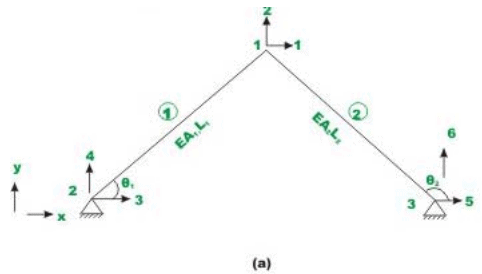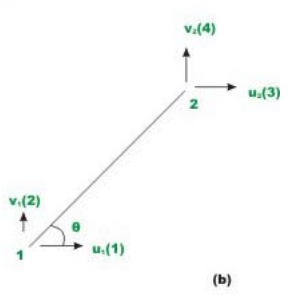Fig 24.11 Analysis of plane - truss

The area of cross-section of the members, its length and its inclination with the x - axis are also shown. Now the member stiffness matrix in the global co- ordinate system for both the members are given by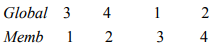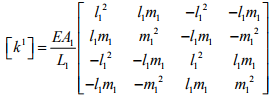(24.21a)

On the member stiffness matrix the corresponding member degrees of freedom and global degrees of freedom are also shown.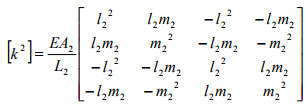(24.21b)

Note that the member stiffness matrix in global co-ordinate system is derived referring to Fig. 24.11b. The node 1 and node 2 remain same for all the members. However in the truss, for member 1, the same node (i.e. node 1 and 2 in Fig. 24.11b) are referred by 2 and 1 respectively. Similarly for member 2, the nodes 1 and 2 are referred by nodes 3 and 4 in the truss. The member stiffness matrix is of the order 4×4. However the truss has six possible displacements and hence truss stiffness matrix is of the order 6×6 . Now it is required to put elements of the member stiffness matrix of the entire truss. The stiffness matrix of the entire truss is known as assembled stiffness matrix. It is also known as structure stiffness matrix; as overall stiffness matrix. Thus, it is clear that by algebraically adding the above two stiffness matrix we get global stiffness matrix. For example the element k111 of the member stiffness matrix of member 1 must go to location (3,3) in the global stiffness matrix. Similarly k211 must go to location (3,3) in the global stiffness matrix. The above procedure may be symbolically written as,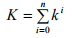(24.22)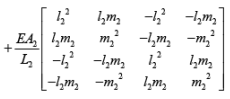The assembled stiffness matrix is of the order 6×6 . Hence, it is easy to visualize assembly if we expand the member stiffness matrix to 6×6 size. The missing columns and rows in matrices k1 and k2 are filled with zeroes. Thus,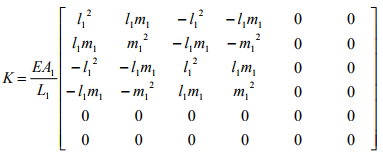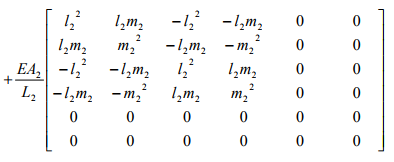(24.24)

Adding appropriate elements of first matrix with the appropriate elements of the second matrix,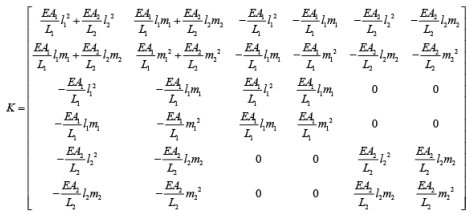If more than one member meet at a joint then the stiffness coefficients of member stiffness matrix corresponding to that joint are added.

After evaluating global stiffness matrix of the truss, the load displacement equation for the truss is written as,

{p} = [K] {u}                                                (24.26)

where {p} is the vector of joint loads acting on the truss, {u} is the vector of joint displacements and  is the global stiffness matrix. The above equation is known as the equilibrium equation. It is observed that some joint loads are known and some are unknown. Also some displacements are known due to support conditions and some displacements are unknown. Hence the above equation may be partitioned and written as,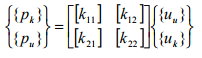(24.27)

where {pk}, {uk} denote vector of known forces and known displacements respectively. And {pu}, {uu} denote vector of unknown forces and unknown displacements respectively.

Expanding equation 24.27,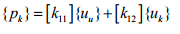(24.28a)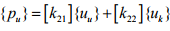(24.28b)

In the present case (vide Fig. 24.11a) the known displacements are u3, u4, u5 and u6. The known displacements are zero due to boundary conditions. Thus, {uk} = {0}. And from equation (24.28a),

{pk} = [k11]{uu}                                    (24.29)

Solving {uu} = [k11]-1 {pk}

where [k11] corresponding to stiffness matrix of the truss corresponding to unconstrained degrees of freedom. Now the support reactions are evaluated from equation (24.28b).

{pu} = [k21]{uu}                                   (24.30)

The member forces are evaluated as follows. Substituting equation (24.10b) {u'} = [T]  {u} in equation (24.6b) {p'} = [k'] {u'}, one obtains

{p'} = [k'] {u'}                      (24.31)

Expanding this equation,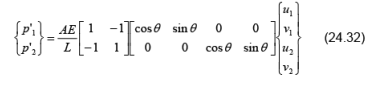Example 24.1
Analyse the two member truss shown in Fig. 24.12a. Assume EA to be constant for all members. The length of each member is 5m.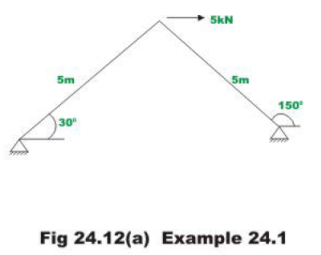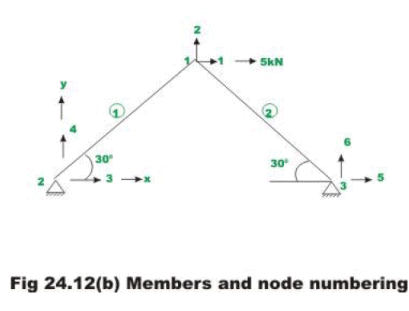The co-ordinate axes, the number of nodes and members are shown in Fig.24.12b. The degrees of freedom at each node are also shown. By inspection it is clear that the displacement u3 = u4 = u5 = u6 = 0. Also the external loads are

p1 = 5 kN  ; p2 = 0 kN.                             (1)

Now member stiffness matrix for each member in global co-ordinate system is (θ1 = 30°)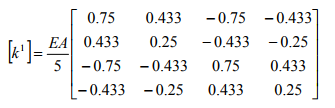(2)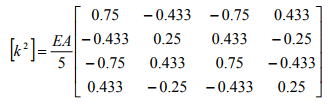.              (3)

The global stiffness matrix of the truss can be obtained by assembling the two member stiffness matrices. Thus,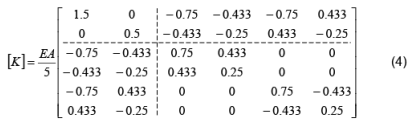Again stiffness matrix for the unconstrained degrees of freedom is,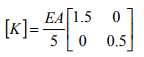(5)

Writing the load displacement-relation for the truss for the unconstrained degrees of freedom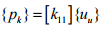(6)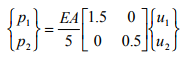(7)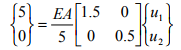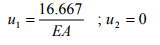(8)

Support reactions are evaluated using equation (24.30).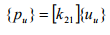(9)

Substituting appropriate values in equation (9),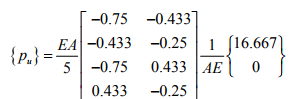(10)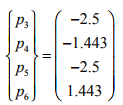(11)

The answer can be verified by equilibrium of joint 1. Also,

p3 + p5 + 5 = 0

Now force in each member is calculated as follows,

Member 1: l = 0.866 ; m = 0.5 ; L = 5m.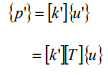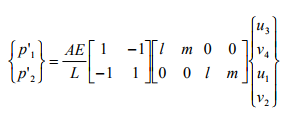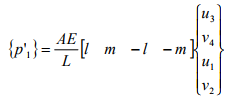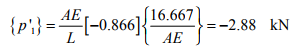Member 2: l −= 0.866 ; m = 0.5 ; L = 5m .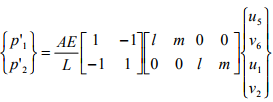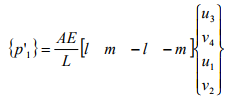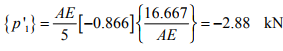Summary

The member stiffness matrix of a truss member in local co-ordinate system is defined. Suitable transformation matrices are derived to transform displacements and forces from the local to global co-ordinate system. The member stiffness matrix of truss member is obtained in global co-ordinate system by suitable transformation. The system stiffness matrix of a plane truss is obtained by assembling member matrices of individual members in global co-ordinate system. In the end, a few plane truss problems are solved using the direct stiffness matrix approach.

Offer running on EduRev: Apply code STAYHOME200 to get INR 200 off on our premium plan EduRev Infinity!

## Structural Analysis

30 videos|122 docs|28 tests

,

,

,

,

,

,

,

,

,

,

,

,

,

,

,

,

,

,

,

,

,

;